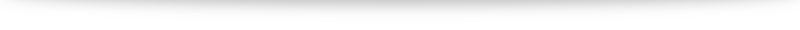# Proofs of common number properties

I will name some properties I will use for future references with MP (my proposition) below:

### (MP1.1) 0 times anything is 0

Let $a$ be any number. Let’s start with $a \cdot 0$. By the property of $0$, we have $a \cdot 0 = a \cdot (0 + 0)$. Using the distributive property gives us $a \cdot 0 + a \cdot 0$. Combining it all together we have $a\cdot 0 = a\cdot 0 + a\cdot 0$. But the definition of $0$ is such that it doesn’t change a number after addition. So $a \cdot 0 = 0$.

### (MP1.2) $(-a)b = – (ab)$

Note the meaning of $-a$ is the additive inverse of $a$, meaning that $a + (-a) = 0$ while the meaning of $-(ab)$ is the additive inverse of $ab$. Let’s add $ab$ with $(-a)b$.
$ab + (-a)b = (a + (-a)) \cdot b$ by the commutative and distributive laws. Since $-a$ is the additive inverse of $a$, this gives us $0 \cdot b$ which is $0$ from MP1.1. Thus $(-a)b$ is the additive inverse of $ab$ which is what we want to prove.

### (MP1.3) $(-a)(-b) = ab$

$(-a)(-b) + -(ab) = (-a)(-b) + (-a)(b)$ by the MP1.2. By the distributive property, $(-a)(-b) + (-a)b = (-a)(-b + b) = (-a)0 = 0$ by MP1.1. Since $-(ab)$ is the additive inverse of $ab$, $(-a)(-b)$ must have been $ab$.

### We cannot have the multiplicative inverse of 0

Suppose there exist the multiplicative inverse of $0$, which we will denote by $0^{-1}$. Then $0 \cdot 0^{-1} = 1$. But by MP1.1, $0$ times anything is 0 so $0=1$. This brings us a whole host of problems: $1 + 1 = 0 + 0 = 0$. So no matter what we do, every number we try to create will all become 0. Hence allowing the multiplicative inverse of 0 means that we can only work with one number: not a very interesting proposition. Conversely, to have more than one number to work with, we cannot allow the multiplicative inverse of 0.

### (MP1.5) If $ab=0$, then $a=0$ or $b=0$.

Case 1: $a=0$. That is part of the solution.
Case 2: $a \neq 0$. Then the multiplicative inverse $a^{-1}$ exists. So $ab= 0$ means $a^{-1} ab = a^{-1} 0$ so $b=0$.

### (MP1.6) $(ab)^{-1} = a^{-1}b^{-1}$

$ab (a^{-1}b^{-1}) = a a^{-1} b b^{-1}$ by the commutative and associative properties which gets us $1 \cdot 1 = 1$ by the property of the multiplicative identity $1$. Hence $a^{-1}b^{-1}$ is the multiplicative inverse of $ab$.